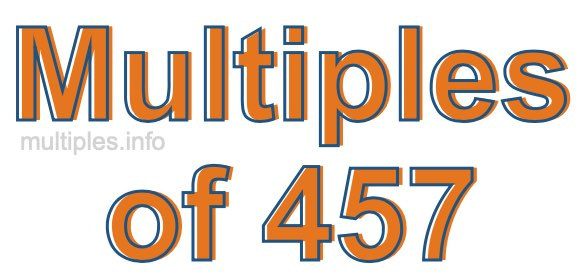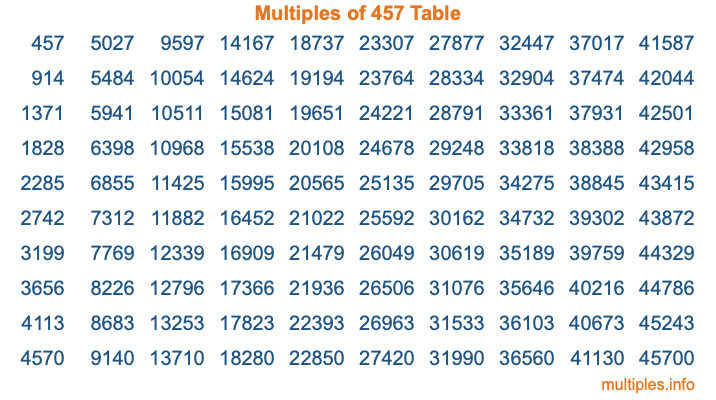Multiples of 457Welcome to the Multiples of 457 page. Here we will first teach you everything you will ever need to know about the multiples of 457, and then give you a study guide summary of everything we taught you to make sure you remember it all. Use this page to look up facts and learn information about the multiples of 457. This page will make you a multiples of four hundred fifty-seven expert!

Definition of Multiples of 457
Multiples of 457 are all the numbers that when divided by 457 equal an integer. Each of the multiples of 457 are called a multiple. A multiple of 457 is created by multiplying 457 by an integer.

Therefore, to create a list of multiples of 457, you start with 1 multiplied by 457, then 2 multiplied by 457, then 3 multiplied by 457, and so on for as long as you want. Thus, the list of the first five multiples of 457 is 457, 914, 1371, 1828, and 2285. To see a larger list of multiples of 457, see the printable image of Multiples of 457 further down on this page. We also have a category where you can choose any nth multiple of 457.

Multiples of 457 Checker
The Multiples of 457 Checker below checks to see if any number of your choice is a multiple of 457. In other words, it checks to see if there is any number (integer) that when multiplied by 457 will equal your number. To do that, we divide your number by 457. If the the quotient is an integer, then your number is a multiple of 457.

Is  a multiple of 457?

Least Common Multiple of 457 and ...
A Least Common Multiple (LCM) is the lowest multiple that two or more numbers have in common. This is also called the smallest common multiple or lowest common multiple and is useful to know when you are adding our subtracting fractions. Enter one or more numbers below (457 is already entered) to find the LCM.

Check out our LCM Calculator if you need more details about the Least Common Multiple or if you need the LCM for different numbers for adding and subtraction fractions.

nth Multiple of 457
As we stated above, 457 is the first multiple of 457, 914 is the second multiple of 457, 1371 is the third multiple of 457, and so on. Enter a number below to find the nth multiple of 457.

th multiple of 457

Multiples of 457 vs Factors of 457
457 is a multiple of 457 and a factor of 457, but that is where the similarities end. All postive multiples of 457 are 457 or greater than 457. All positive factors of 457 are 457 or less than 457.

Below is the beginning list of multiples of 457 and the factors of 457 so you can compare:

Multiples of 457: 457, 914, 1371, 1828, 2285, etc.

Factors of 457: 1, 457

As you can see, the multiples of 457 are all the numbers that you can divide by 457 to get a whole number. The factors of 457, on the other hand, are all the whole numbers that you can multiply by another whole number to get 457.

It's also interesting to note that if a number (x) is a factor of 457, then 457 will also be a multiple of that number (x).

Multiples of 457 vs Divisors of 457
The divisors of 457 are all the integers that 457 can be divided by evenly. Below is a list of the divisors of 457.

Divisors of 457: 1, 457

The interesting thing to note here is that if you take any multiple of 457 and divide it by a divisor of 457, you will see that the quotient is an integer.

Multiples of 457 Table
Below is an image of the first 100 multiples of 457 in a table. The table is in chronological order, column by column. The first column has the first ten multiples of 457, the second column has the next ten multiples of 457, and so on.The Multiples of 457 Table is also referred to as the 457 Times Table or Times Table of 457. You are welcome to print out our table for your studies.

Negative Multiples of 457
Although not often discussed or needed in math, it is worth mentioning that you can make a list of negative multiples of 457 by multiplying 457 by -1, then by -2, then by -3, and so on, to get the following list of negative multiples of 457:

-457, -914, -1371, -1828, -2285, etc.

Multiples of 457 Summary
Below is a summary of important Multiples of 457 facts that we have discussed on this page. To retain the knowledge on this page, we recommend that you read through the summary and explain to yourself or a study partner why they hold true.

There are an infinite number of multiples of 457.

A multiple of 457 divided by 457 will equal a whole number.

457 divided by a factor of 457 equals a divisor of 457.

The nth multiple of 457 is n times 457.

The largest factor of 457 is equal to the first positive multiple of 457.

457 is a multiple of every factor of 457.

457 is a multiple of 457.

A multiple of 457 divided by a divisor of 457 equals an integer.

457 divided by a divisor of 457 equals a factor of 457.

Any integer times 457 will equal a multiple of 457.

Multiples of a Number
Here you can get the multiples of another number, all with the same attention to detail as we did for multiples of 457 on this page.

Multiples of
Multiples of 458
Did you find our page about multiples of four hundred fifty-seven educational? Do you want more knowledge? Check out the multiples of the next number on our list!